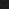JavaPerspective.com  >   Beginner Tutorials  >   3. Java Basics  >   3.6. Java operators examples  >   3.6.3. Conditional and relational operators examples

3.6.3. Conditional and relational operators examples
Last updated: 27 January 2013.

The operators <, <=, >, >=, ==, !=, &&, &, ||, | are very easy to use. So let's add a single method called conditionalAndRelationalOperators to illustrate their uses:

public void conditionalAndRelationalOperators(){

int a = 1;

int b = 2;

System.out.println
(a < b);

System.out.println
(a <= b);

System.out.println
(a > b);

System.out.println
(a >= b);

System.out.println
(a == b);

System.out.println
(a != b);

System.out.println
( (a < b) && (a > 0) );

System.out.println
( (a >= b) & (b < 0) );

System.out.println
( (a < b) || (a > 0) );

System.out.println
( (a >= b) | (b < 0) );
}

Here is the class App:

public class App {

public static void main(String[] args) {

new JavaOperators().conditionalAndRelationalOperators();

}

}

The output is:

true
true
false
false
false
true
true
false
true
false

The difference between the operators && and & is that && does not evaluate the right-hand operand if the left-hand operand is false whereas the operator & always evaluates both operands. If you do not specifically want to evaluate the right-hand operand when the left-hand operand is false, the operator && is the one to use.

Likewise, the operator || does not evaluate the right-hand operand if the left-hand operand is true whereas the operator | always evaluates both operands. So the operator || is the one to use if you do not specifically want to evaluate the right-hand operand when the left-hand operand is true.

You are here :  JavaPerspective.com  >   Beginner Tutorials  >   3. Java Basics  >   3.6. Java operators examples  >   3.6.3. Conditional and relational operators examples
Next tutorial :  JavaPerspective.com  >   Beginner Tutorials  >   3. Java Basics  >   3.6. Java operators examples  >   3.6.4. Bitwise operators examples